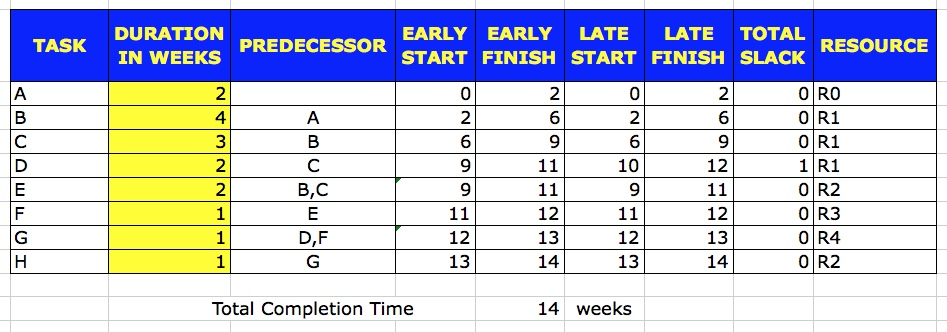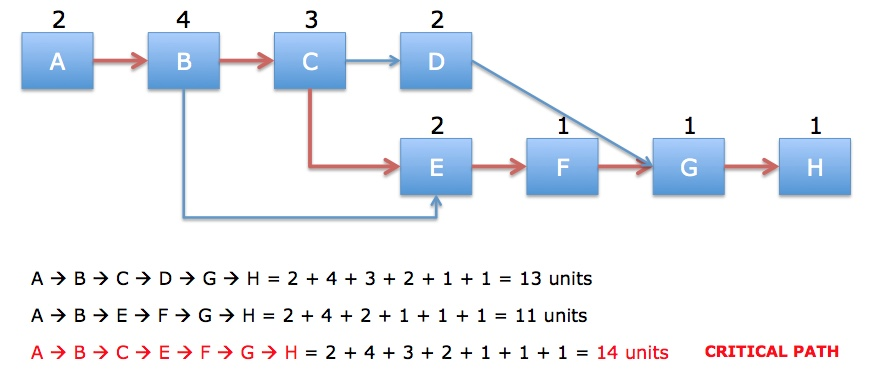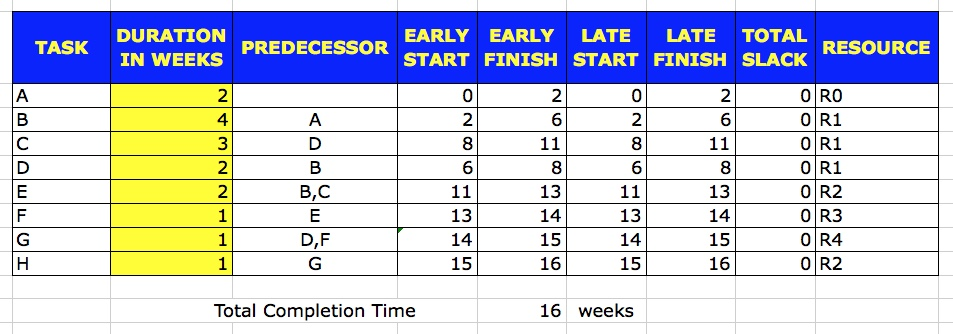Technical

# Minimum Slack Rule

Most Projects undergo resource crunch. This is especially true for IT Projects. Resource crunch can occur due to various reasons like the same resource has the expertise for multiple tasks of the project, OR a resource assigned to the Project may be required in another Project, OR an assigned resource leaves the company, etc.

To deal with situations like this, the Project Manager or the Project Planner needs alter the Project Schedule as originally planned. To alter the Project Schedule under these circumstances in a scientific way, we can use the Minimum Slack Rule. I demonstrate it below.

Suppose, we start with a Project Schedule as provided in the picture below.The Early Start and Early Finish is calculated using the Forward Pass. The Late Start and Late Finish is calculated using the Backward Pass.

The Total Slack is calculated as follows:

```Total Slack = Late Finish - Early Finish = Late Start - Early Start ```

The network diagram for this Plan is as shown below.With normal duration for each task, the project completion time is 11 weeks. The critical part is shown in red in the diagram.

Tasks on the Critical Path always have a Total Slack of 0.

Now, let us allocate resources to the Project as shown below.We notice that Tasks B, C and D require the resource R1. Consider this to be a constraint on the Project. However, we wanted to start Tasks B, C and D simultaneously. However, due to this constraint, this would not be possible. We need to decide how we will sequence the Tasks B, C and D so that resource R1 can work on them.

According to the Minimum Slack Rule, when there is a contest between tasks, we should first take the task which has the minimum Total Slack.

If we apply this rule, then we should take up task B first as it has a Total Slack of 0. Next, we should take up task C as it has a Total Slack of 1. And we should take up task D last among these 3 as it has a Total Slack of 5.

If we consider this plan, let us see what the Project Plan looks like.The network diagram for this plan is as shown below.So, we see that under this resource constraint, if we apply Minimum Slack Rule, we can complete the Project in 14 weeks.

Let us validate this. Now, let us alter the task order such that we conduct task D before task C. The Plan would look as shown below.The network diagram for this plan is shown below.You can see that the Project Duration is now 16 weeks, which is greater than 14 weeks when we applied Minimum Slack Rule.